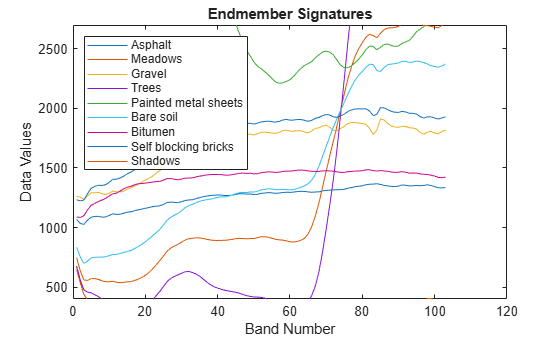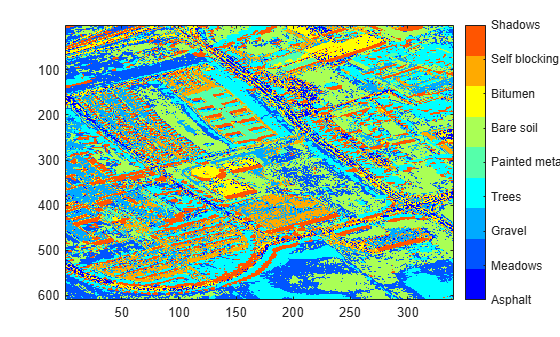# Hyperspectral Image Analysis Using Maximum Abundance Classification

This example shows how to identify different regions in a hyperspectral image by performing maximum abundance classification (MAC). An abundance map characterizes the distribution of an endmember across a hyperspectral image. Each pixel in the image is either a pure pixel or a mixed pixel. The set of abundance values obtained for each pixel represents the percentage of each endmembers present in that pixel. In this example, you will classify the pixels in a hyperspectral image by finding the maximum abundance value for each pixel and assigning it to the associated endmember class.

This example requires the Image Processing Toolbox™ Hyperspectral Imaging Library. You can install the Image Processing Toolbox Hyperspectral Imaging Library from Add-On Explorer. For more information about installing add-ons, see Get and Manage Add-Ons. The Image Processing Toolbox Hyperspectral Imaging Library requires desktop MATLAB®, as MATLAB® Online™ and MATLAB® Mobile™ do not support the library.

This example uses a data sample from the Pavia University dataset as test data. The test data contains nine endmembers that represent these ground truth classes: Asphalt, Meadows, Gravel, Trees, Painted metal sheets, Bare soil, Bitumen, Self blocking bricks, and Shadows.

Load the `.mat` file containing the test data into the workspace. The `.mat` file contains an array `paviaU`, representing the hyperspectral data cube and a matrix `signatures`, representing the nine endmember signatures taken from the hyperspectral data. The data cube has 103 spectral bands with wavelengths ranging from 430 nm to 860 nm. The geometric resolution is 1.3 meters and the spatial resolution of each band image is 610-by-340.

```load('paviaU.mat'); img = paviaU; sig = signatures;```

Compute the central wavelength for each spectral band by evenly spacing the wavelength range across the number of spectral bands.

```wavelengthRange = [430 860]; numBands = 103; wavelength = linspace(wavelengthRange(1),wavelengthRange(2),numBands);```

Create a `hypercube` object using the hyperspectral data cube and the central wavelengths. Then estimate an RGB image from the hyperspectral data. Set the `ContrastStretching` parameter value to `true` in order to improve the contrast of the RGB output. Visualize the RGB image.

```hcube = hypercube(img,wavelength); rgbImg = colorize(hcube,'Method','RGB','ContrastStretching',true); figure imshow(rgbImg)```The test data contains the endmember signatures of nine ground truth classes. Each column of `sig` contain the endmember signature of a ground truth class. Create a table that lists the class name for each endmember and the corresponding column of `sig`.

```num = 1:size(sig,2); endmemberCol = num2str(num'); classNames = {'Asphalt';'Meadows';'Gravel';'Trees';'Painted metal sheets';'Bare soil';... 'Bitumen';'Self blocking bricks';'Shadows'}; table(endmemberCol,classNames,'VariableName',{'Column of sig';'Endmember Class Name'})```
```ans=9×2 table Column of sig Endmember Class Name _____________ ________________________ 1 {'Asphalt' } 2 {'Meadows' } 3 {'Gravel' } 4 {'Trees' } 5 {'Painted metal sheets'} 6 {'Bare soil' } 7 {'Bitumen' } 8 {'Self blocking bricks'} 9 {'Shadows' } ```

Plot the endmember signatures.

```figure plot(sig) xlabel('Band Number') ylabel('Data Values') ylim([400 2700]) title('Endmember Signatures') legend(classNames,'Location','NorthWest')```### Estimate Abundance Maps

Create abundance maps the endmembers by using the `estimateAbundanceLS` function and select the method as full constrained least squares (FCLS). The function outputs the abundance maps as a 3-D array with the spatial dimensions as the input data. Each channel is the abundance map of the endmember from the corresponding column of signatures. In this example, the spatial dimension of the input data is 610-by-340 and the number of endmembers is 9. So, the size of the output abundance map is 610-by-340-by-9.

`abundanceMap = estimateAbundanceLS(hcube,sig,'Method','fcls');`

Display the abundance maps.

```fig = figure('Position',[0 0 1100 900]); n = ceil(sqrt(size(abundanceMap,3))); for cnt = 1:size(abundanceMap,3) subplot(n,n,cnt) imagesc(abundanceMap(:,:,cnt)) title(['Abundance of ' classNames{cnt}]) hold on end hold off```### Perform Maximum Abundance Classification

Find the channel number of the largest abundance value for each pixel. The channel number returned for each pixel corresponds to the column in `sig` that contains the endmember signature associated with the maximum abundance value of that pixel. Display a color coded image of the pixels classified by maximum abundance value.

```[~,matchIdx] = max(abundanceMap,[],3); figure imagesc(matchIdx) colormap(jet(numel(classNames))) colorbar('TickLabels',classNames)```Segment the classified regions and overlay each of them on the RGB image estimated from the hyperspectral data cube.

```segmentImg = zeros(size(matchIdx)); overlayImg = zeros(size(abundanceMap,1),size(abundanceMap,2),3,size(abundanceMap,3)); for i = 1:size(abundanceMap,3) segmentImg(matchIdx==i) = 1; overlayImg(:,:,:,i) = imoverlay(rgbImg,segmentImg); segmentImg = zeros(size(matchIdx)); end```

Display the classified and the overlaid hyperspectral image regions along with their class names. From the images, you can see that the asphalt, trees, bare soil, and brick regions have been accurately classified.

```figure('Position',[0 0 1100 900]); n = ceil(sqrt(size(abundanceMap,3))); for cnt = 1:size(abundanceMap,3) subplot(n,n,cnt); imagesc(uint8(overlayImg(:,:,:,cnt))); title(['Regions Classified as ' classNames{cnt}]) hold on end hold off```